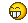# logical puzzle III

#### dfx

Registered
well it's really more like a mathematical puzzle... it's kinda similar to the other one ( http://forum.ah.fm/mellow-lounge/12423-logical-puzzle-ii.html ), so good luckthe story is about harry. harry wants to be rich. he's dreaming about winning the lottery. and one day, there's suddenly a bright flash in his room, and a fairy appears.

of course, the fairy grants him a wish, and of course, harry asks her for the lottery numbers. but the fairy replies, "what, all six numbers? no no, i can't do that, that would be six wishes, not only just one!"

however, the fairy still writes a number on a piece of paper, and she's telling harry "if you sum up all six numbers from the next lottery drawing, you will get this number here as result".

harry looks at the number and desperately says "omg, there's gotta be thousands of combinations of six numbers between 1 and 49 that yield this number as sum". so the fairy gives him another hint: "why don't you figure out how many combinations there are exactly to yield this sum? then, if you take this number and multiply it with the number on the paper, you get a very large number of several millions, and this is exactly the same number you will get when multiplying all six lottery numbers from the drawing with each other!"

and poof, the fairy disappears. harry starts calculating. and lo and behold, he gets all six numbers at the next lottery drawing.

which are the six numbers from the drawing?

Last edited:There goes another day's work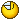There goes another day's work:LMAO:

Mine too...

Do we get to know what the sum the fairy handed him is?

Do we get to know what the sum the fairy handed him is?

of course notof course notD'oh! hehe...

any progress yet?any progress yet?Yeah, kind of... that dumbass Harry should ask the Fairy to give him money instead of the numbers - feck I'm smart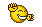- Numbers are 1-9, so highest possible number on the paper should be 9x6=54 (9+9+9+9+9+9). 49+(5x1)=54... Number on paper is 54!

- 9^6 = 531,441 possibilities

- combinations x number on paper = millions = nr1xn2xnr3xnr4xnr5xnr6

- number on paper = (nr1+nr2+nr3+nr4+nr5+nr6) = 54

- So, combinations x (nr1+nr2+nr3+nr4+nr5+nr6)= millions = nr1xn2xnr3xnr4xnr5xnr6
Gives 531,441 x 54 = 28,697,814 = nr1xn2xnr3xnr4xnr5xnr6

Ah fack... my results wer ebetter before I edited this post... I'm out

Last edited:
well, if you're working with 1-9, the highest possible number on paper would be 4+5+6+7+8+9 = 39.

and the number of combinations isn't 9^6, but rather ( 9! / ( 6! * (9 - 6)! )) = 84.

but if you're working with the actual 49, you get 279 as the highest possible number on the paper, and the number of combinations is 13,983,816.

well, if you're working with 1-9, the highest possible number on paper would be 4+5+6+7+8+9 = 39.

and the number of combinations isn't 9^6, but rather ( 9! / ( 6! * (9 - 6)! )) = 84.

but if you're working with the actual 49, you get 279 as the highest possible number on the paper, and the number of combinations is 13,983,816.

Errrr a 6 number lottery-number... could be 9-9-9-9-9-9= 54! Where did you get 4+5+6+7+8+9 = 39??????

And the rest is nutz aswell plz explain better...?

Errrr a 6 number lottery-number... could be 9-9-9-9-9-9= 54! Where did you get 4+5+6+7+8+9 = 39??????

And the rest is nutz aswell plz explain better...?

because if you're working with 1-9, the 9 can only be drawn once - after that, the highest possible number is 8, and so on.

same goes for 1-49. 49 is drawn first, then it's out of the pot, so the highest possible number for the second drawn number is 48, etc.

you know how lottery works, right?

Man there are so many lotteries... So this is the oldschool lottery with 49 balls in a bucket... Got it now thnx!

:bump:

Yeah, kind of... that dumbass Harry should ask the Fairy to give him money instead of the numbers - feck I'm smartAndy wins at logic.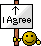This one actually requires some deep math.. I can't do this one. D=

Andy wins at logic.I win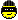44,45,46,47,48,49 ???

he should of wished for more wishes